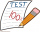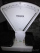# Fraction calculator

The calculator performs basic and advanced operations with fractions, expressions with fractions combined with integers, decimals, and mixed numbers. It also shows detailed step-by-step information about the fraction calculation procedure. Solve problems with two, three, or more fractions and numbers in one expression.

## Result:

### 32/3 × 21/7 = 55/7 = 7 6/7 ≅ 7.8571429

Spelled result in words is fifty-five sevenths (or seven and six sevenths).

### How do you solve fractions step by step?

1. Conversion a mixed number 3 2/3 to a improper fraction: 3 2/3 = 3 2/3 = 3 · 3 + 2/3 = 9 + 2/3 = 11/3

To find new numerator:
a) Multiply the whole number 3 by the denominator 3. Whole number 3 equally 3 * 3/3 = 9/3
b) Add the answer from previous step 9 to the numerator 2. New numerator is 9 + 2 = 11
c) Write a previous answer (new numerator 11) over the denominator 3.

Three and two thirds is eleven thirds
2. Conversion a mixed number 2 1/7 to a improper fraction: 2 1/7 = 2 1/7 = 2 · 7 + 1/7 = 14 + 1/7 = 15/7

To find new numerator:
a) Multiply the whole number 2 by the denominator 7. Whole number 2 equally 2 * 7/7 = 14/7
b) Add the answer from previous step 14 to the numerator 1. New numerator is 14 + 1 = 15
c) Write a previous answer (new numerator 15) over the denominator 7.

Two and one seventh is fifteen sevenths
3. Multiple: 11/3 * 15/7 = 11 · 15/3 · 7 = 165/21 = 55 · 3/7 · 3 = 55/7
Multiply both numerators and denominators. Result fraction keep to lowest possible denominator GCD(165, 21) = 3. In the next intermediate step, , cancel by a common factor of 3 gives 55/7.
In words - eleven thirds multiplied by fifteen sevenths = fifty-five sevenths.

#### Rules for expressions with fractions:

Fractions - use the slash “/” between the numerator and denominator, i.e., for five-hundredths, enter 5/100. If you are using mixed numbers, be sure to leave a single space between the whole and fraction part.
The slash separates the numerator (number above a fraction line) and denominator (number below).

Mixed numerals (mixed fractions or mixed numbers) write as non-zero integer separated by one space and fraction i.e., 1 2/3 (having the same sign). An example of a negative mixed fraction: -5 1/2.
Because slash is both signs for fraction line and division, we recommended use colon (:) as the operator of division fractions i.e., 1/2 : 3.

Decimals (decimal numbers) enter with a decimal point . and they are automatically converted to fractions - i.e. 1.45.

The colon : and slash / is the symbol of division. Can be used to divide mixed numbers 1 2/3 : 4 3/8 or can be used for write complex fractions i.e. 1/2 : 1/3.
An asterisk * or × is the symbol for multiplication.
Plus + is addition, minus sign - is subtraction and ()[] is mathematical parentheses.
The exponentiation/power symbol is ^ - for example: (7/8-4/5)^2 = (7/8-4/5)2

#### Examples:

subtracting fractions: 2/3 - 1/2
multiplying fractions: 7/8 * 3/9
dividing Fractions: 1/2 : 3/4
exponentiation of fraction: 3/5^3
fractional exponents: 16 ^ 1/2
adding fractions and mixed numbers: 8/5 + 6 2/7
dividing integer and fraction: 5 ÷ 1/2
complex fractions: 5/8 : 2 2/3
decimal to fraction: 0.625
Fraction to Decimal: 1/4
Fraction to Percent: 1/8 %
comparing fractions: 1/4 2/3
multiplying a fraction by a whole number: 6 * 3/4
square root of a fraction: sqrt(1/16)
reducing or simplifying the fraction (simplification) - dividing the numerator and denominator of a fraction by the same non-zero number - equivalent fraction: 4/22
expression with brackets: 1/3 * (1/2 - 3 3/8)
compound fraction: 3/4 of 5/7
fractions multiple: 2/3 of 3/5
divide to find the quotient: 3/5 ÷ 2/3

The calculator follows well-known rules for order of operations. The most common mnemonics for remembering this order of operations are:
PEMDAS - Parentheses, Exponents, Multiplication, Division, Addition, Subtraction.
BEDMAS - Brackets, Exponents, Division, Multiplication, Addition, Subtraction
BODMAS - Brackets, Of or Order, Division, Multiplication, Addition, Subtraction.
GEMDAS - Grouping Symbols - brackets (){}, Exponents, Multiplication, Division, Addition, Subtraction.
Be careful, always do multiplication and division before addition and subtraction. Some operators (+ and -) and (* and /) has the same priority and then must evaluate from left to right.

## Fractions in word problems:

• Equivalent expressionsA coach took his team out for pizza after their last game. There were 14 players, so they had to sit in smaller groups at different tables. Six players sat at one table and got 4 small pizzas to share equally. The other players sat at the different table
• ArrangeArrange the following in descending order: 0.32, 2on5, 27%, 1 on 3
• Ten fractionsWrite ten fractions between 1/3 and 2/3
• SandySandy, John and Marg baked pies for the Bake Sale. Sandy cut his pies into 6ths, John but his into 8ths and Marg cut hers into quarters. Sandy sold 11/6, John sold  1 3/8 pies and Marg sold 9/4 pies. Who sold the most pies? Who sold the fewest?Rhea answered 5/11 in the questions correctly and Precious answered 7/11 of it correctly. If each problem is worth the same amount, who got the higher score?
• Engineer KažimírThe difference between politicians-demagogues and reasonable person with at least primary education beautifully illustrated by the TV show example. "Engineer" Kažimír says that during their tenure there was a large decline in the price of natural gas, pri
• TorqueTorque and Mari each multiplied 1/8 inch times 5/8 inch. Tartaric 5/8 squares point inches. And Marie got 5/64 squared thought inches tall. Which student found a corrupt area?
• SayavongSayavong is making cookies for the class. He has a recipe that calls for 3 and 1/2 cups of flour. He has 7/8 of a cup of wheat flour, and 2 and 1/2 cups of white flour. Does Mr. Sayavong have enough flour to make the cookies?
• A laundryMr. Green washed 1/4 of his laundry. His son washed 3/7 of it. Who washed most of the laundry? How much of the laundry still needs to be washed?
• TroopsThe route is long 147 km and the first-day first regiment went at an average speed of 12 km/h and journey back 21 km/h. The second day went second regiment the same route at an average speed of 22 km/h there and back. Which regiment will take route longer
• Comparing and sortingArrange in descending order this fractions: 2/7, 7/10 & 1/2
• Pizza palaceJosh is at Enzo's pizza palace. He can sit at a table with 5 of his friends or at a different table with seven of his friends. The same size pizza is shared equally among the people at each table. At which table should Josh sit to get more pizza? (write a
• Evaluate mixed expressionsWhich of the following is equal to 4 and 2 over 3 divided by 3 and 1 over 2? A. 4 and 2 over 3 times 3 and 2 over 1 B. 14 over 3 times 2 over 7 C. 14 over 3 times 7 over 2 D. 42 over 3 times 2 over 31